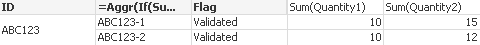# QlikView App Dev

Discussion Board for collaboration related to QlikView App Development.

Announcements
Skip the ticket, Chat with Qlik Support instead for instant assistance.
cancel
Showing results for
Did you mean:Not applicable

## Use an expression in a calculated dimension

Hi everyone ,

I hope you can help me with this issue.

I have this data :

 ID ID2 Quantity1 Quantity2 Flag ABC123 ABC123-1 10 15 Validated ABC123 ABC123-2 10 12 Validated ABC123 ABC123-3 15 15 Validated

I tried to have a table to show only lines where the sum of quantity1 < the sum of quantity2 when flag="Validated".

I have variables like this :

v_quantity1 = sum({<Flag={'Validated'}>} quantity1)

v_quantity2 = sum({<Flag={'Validated'}>} quantity2)

I tried to use a calculated dimension like this :

- Test 1 : if(\$(v_quantity1) < \$(v_quantity2),1,null()) + check the box "suppress when the values is null"

- Test 2 : if( if(Flag="Validated",sum(quantity1) < if(Flag="Validated",sum(quantity2),1,null()) + check the box "suppress when the values is null"

It doesn't work. I have this error : "Garbage after expression"

I simplify my issue but I have to do a sum. I have others filters like X = "1" for example and it works in a calculated dimension.

Do you have any idea please ?

Kind regards ,

Gwendaline

1 Solution

Accepted SolutionsMaster III

Hi,

maybe this

Dimensions :

ID

Aggr(If(Sum(Quantity1) < Sum(Quantity2) and Flag = 'Validated',ID2),ID2)

FlagRegards,

Antonio

3 RepliesMaster III

Hi,

maybe this

Dimensions :

ID

Aggr(If(Sum(Quantity1) < Sum(Quantity2) and Flag = 'Validated',ID2),ID2)

FlagRegards,

AntonioNot applicable
Author

Yes, it works !

Thank you Antonio !Contributor

Hello,

Maybe, somebody faced this problem and could help me :-).

I created the calculated column. After the receiving the results, I need remove "Null"s

"Expressions" returns me the correct results, but impossible remove Null values in model

Calculated dimension, where Null remove is possible, - presents not correct results .

my calculation is:

=if (T_Number = Max_T_Number or (IsNull (T_Number) and Qty_On_Hand > 0.1 ), 'New', Null())

 Number T_Number Max_T_Number Expressions Calculated dimension Qty_On_Hand ABC1 New 1 ABC2 T119565 T119566 New New ABC3 New 1 ABC4 T119568 T119568 New New ABC5

thank you!Community Browser